# Got stuck at a point -2

While working with algebra, I got a very interesting equation to solve which is as follows

$a^y=y$ $\Rightarrow a^{a^{y}}=y$ You may continue like this and you will get $\boxed{\red{a^{a^{a^{a^{a...}}}}=y}}$ Where $$a$$ is known, $$a∈(0,e^{\frac{1}{e}}]$$, can you solve for $$y$$ such that $$y∈(0,e]$$?

Any solution will be appreciatedNote by Zakir Husain
1 year, 1 month ago

This discussion board is a place to discuss our Daily Challenges and the math and science related to those challenges. Explanations are more than just a solution — they should explain the steps and thinking strategies that you used to obtain the solution. Comments should further the discussion of math and science.

When posting on Brilliant:

• Use the emojis to react to an explanation, whether you're congratulating a job well done , or just really confused .
• Ask specific questions about the challenge or the steps in somebody's explanation. Well-posed questions can add a lot to the discussion, but posting "I don't understand!" doesn't help anyone.
• Try to contribute something new to the discussion, whether it is an extension, generalization or other idea related to the challenge.

MarkdownAppears as
*italics* or _italics_ italics
**bold** or __bold__ bold
- bulleted- list
• bulleted
• list
1. numbered2. list
1. numbered
2. list
Note: you must add a full line of space before and after lists for them to show up correctly
paragraph 1paragraph 2

paragraph 1

paragraph 2

[example link](https://brilliant.org)example link
> This is a quote
This is a quote
    # I indented these lines
# 4 spaces, and now they show
# up as a code block.

print "hello world"
# I indented these lines
# 4 spaces, and now they show
# up as a code block.

print "hello world"
MathAppears as
Remember to wrap math in $$ ... $$ or $ ... $ to ensure proper formatting.
2 \times 3 $2 \times 3$
2^{34} $2^{34}$
a_{i-1} $a_{i-1}$
\frac{2}{3} $\frac{2}{3}$
\sqrt{2} $\sqrt{2}$
\sum_{i=1}^3 $\sum_{i=1}^3$
\sin \theta $\sin \theta$
\boxed{123} $\boxed{123}$

Sort by:

This property or in fact this question is known as tetration.

Interestingly if we let

$a^{a^{a^{a^{a^{}}}}} = 2$ $a^2 = 2 \implies a = \sqrt{2}$

And again

$b^{b^{b^{b^{b^{}}}}} = 4$ $b^4 = 4 \implies b = \sqrt{2}$

Now, since $a=b$, $\implies a^{a^{a^{a^{a^{}}}}} = b^{b^{b^{b^{b^{}}}}} \implies 2=4$?

Does this mean $2 = 4$?

- 1 year, 1 month ago

Aren't those two two different equations. So, you can't equate both of them, ain't it?

- 1 year, 1 month ago

Rethink, i have edited my comment a bit

- 1 year, 1 month ago

- 1 year, 1 month ago

well all values of $a$ where $a∈(1,e^\frac{1}{e})$ follow this behaviour

- 1 year, 1 month ago

Yes

- 1 year, 1 month ago

@Mahdi Raza - It is also a math failure, a potential Math also fails! -3. Well, what could be it's explanation?

- 1 year, 1 month ago

I remember seeing this question somewhere. The answer said that it is limited for some values of $a$ or $b$, but I don't remember much.

- 1 year, 1 month ago

This might help :)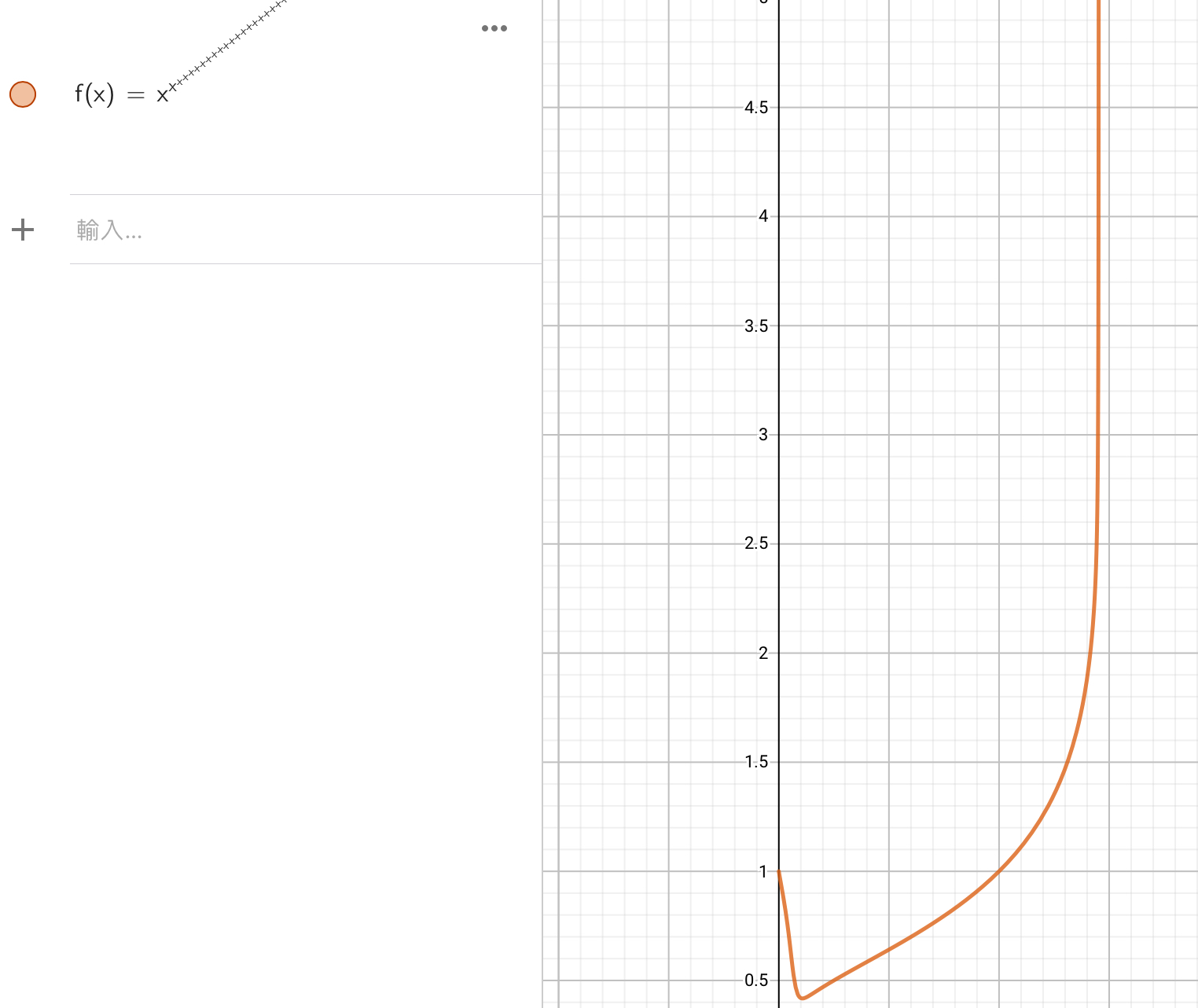The number of x’s are finite, yet the graph starts to approach a straight line already.

- 1 year, 1 month ago

The straight line at the end is $x = e^{\frac{1}{e}} \approx 1.444667861009766$

- 1 year, 1 month ago

Oh! That is interesting :)

- 1 year, 1 month ago

@Jeff Giff, For infinite $x$ you should graph $y=x^y$ instead of this poor approximation, because this will give you the exact graph.

- 1 year, 1 month ago

Oh, I never thought of that! Silly me :)

- 1 year, 1 month ago

@Jeff Giff, how you put this image in the comment?

- 1 year, 1 month ago

First try posting the image in a question. Then copy the link form. Finally paste it onto the comment :)

- 1 year, 1 month ago

What i do is go to a previous solution, insert media, copy the location code, and paste it in the comments. It is tedious i know

- 1 year, 1 month ago

@Jeff Giff, see the graph below: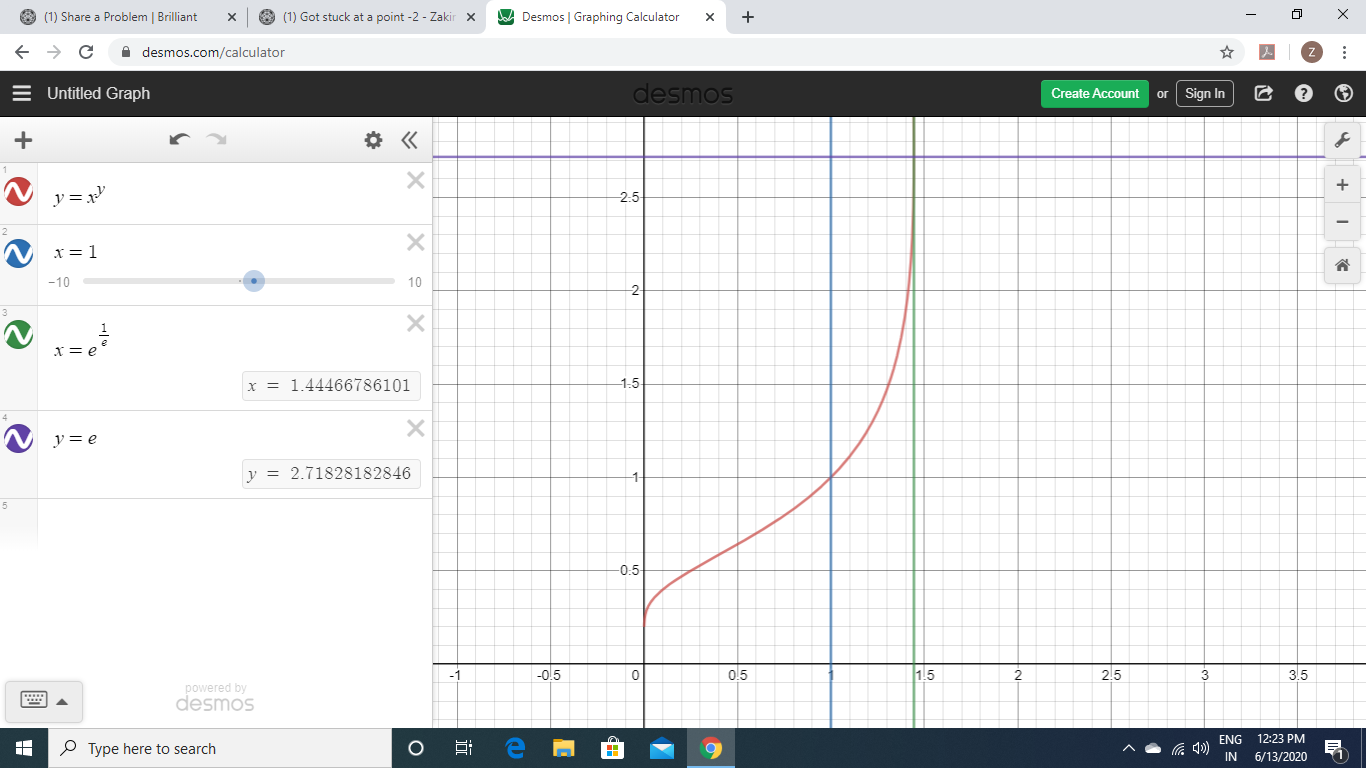I only want a formula which relates all the $x$ and $y$ of the points of $x^y=y$ in the rectangle formed by the $x-axis,y-axis,y=e,x=e^\frac{1}{e}$. As I said in my comment

- 1 year, 1 month ago

Oh. I’ll try to help:)

- 1 year, 1 month ago

Maybe it’s related to $[(x+a)^n+b]\times c$

- 1 year, 1 month ago

@Mahdi Raza- Explanation to the contradiction

Let $x=y^{y^{y^{.^{.^{.}}}}} \Rightarrow x=y^x$ assuming $x ≠ 0$ $y=x^\frac{1}{x}$

Now the maxima of $x^\frac{1}{x}$ is $e^\frac{1}{e}$ and for any $x | x \in (e,∞)$, $y \in (1,e^\frac{1}{e})$.

And for any $x | x \in (1,e)$, $y \in (1,e^\frac{1}{e})$

So for any two variables $x_1$ and $x_2$ $| x_1\in (1,∞),x_2 \in (1,∞)$ and $x_1^\frac{1}{x_1}=x_2^\frac{1}{x_2} \nRightarrow x_1=x_2$

And in your case, applying above statement $a=2^\frac{1}{2}$ and $b=4^\frac{1}{4} \Rightarrow a=b \nRightarrow 2=4$

- 1 year, 1 month ago

• If you haven't read the note above and the comment above yet, then kindly read them first.
@Chew-Seong Cheong, @Justin Travers , @jordi curto , @Yajat Shamji , @Mahdi Raza , @Kumudesh Ghosh , @Alak Bhattacharya , @Vinayak Srivastava , @Aryan Sanghi , @Jeff Giff , @Marvin Kalngan .

Now a new problem arises, if $x_1^\frac{1}{x_1}=x_2^\frac{1}{x_2}; x_1≠x_2$ then what is the relation between $x_1$ and $x_2$ $?$

- 1 year, 1 month ago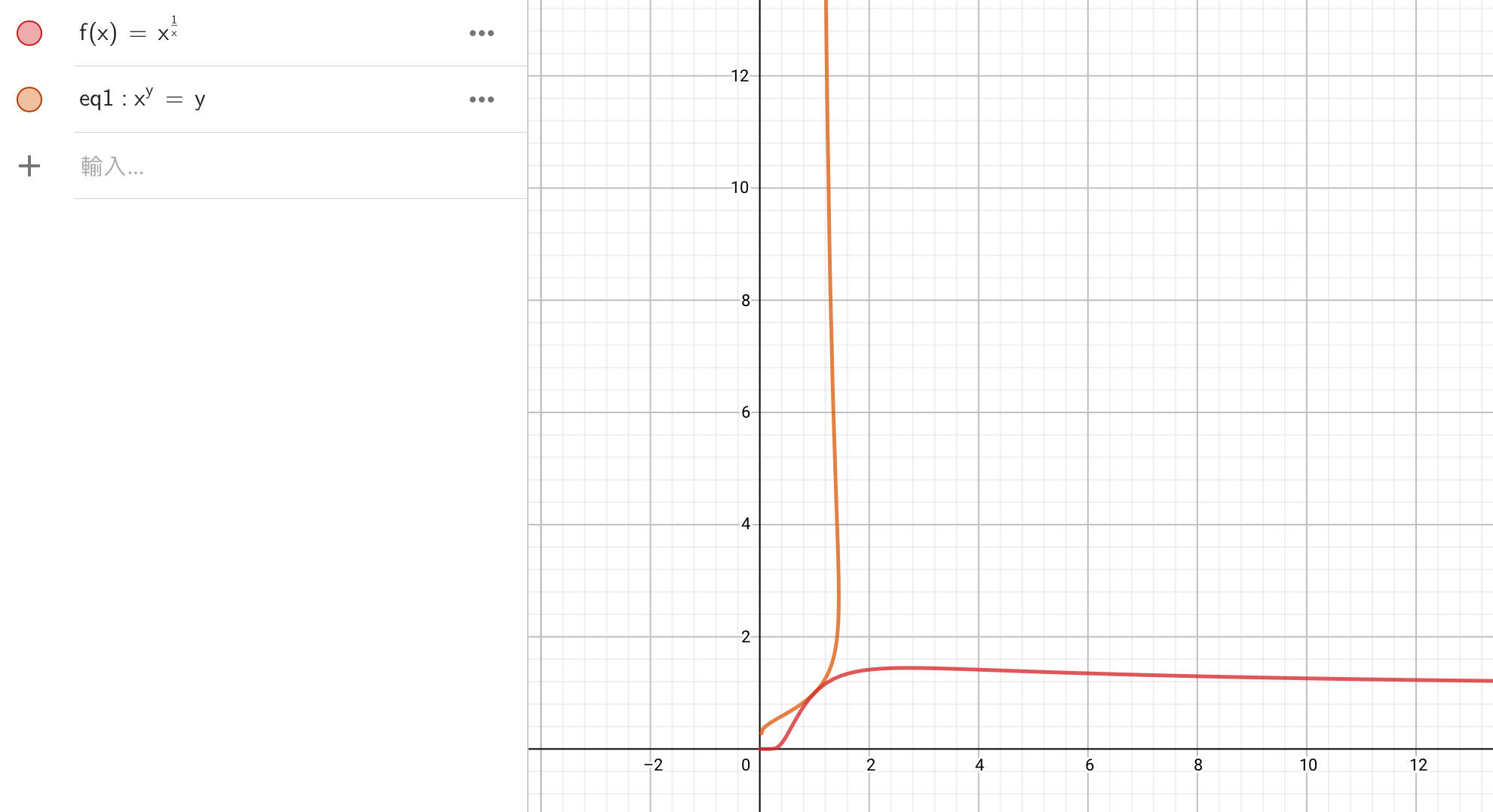This might help :)

- 1 year, 1 month ago

$x_1,x_2$ are the $x$ values of the intersection between $x^{\frac 1x}$ and $y=x_1^{\frac {1}{x_1}}$.

- 1 year, 1 month ago

The graphs are intersecting at $(1,1)$, that is when $x_1=1,x_2=1$, in that case $x_1=x_2$. I'm talking about the case
When $x_1^\frac{1}{x_1}=x^\frac{1}{x_2}$ but $x_1 \neq x_2$. For example when $x_1 = 4; x_2=2$; then $2^\frac{1}{2}=\sqrt{2}=4^\frac{1}{4}$ but $2 \neq 4$

- 1 year, 1 month ago

But the graphs in the pic aren’t the ones that I mentioned:)

- 1 year, 1 month ago

I just meant to say that the graphs in the picture are symmetrical along $x=y.$

- 1 year, 1 month ago

Also, $x_1$ is on the interval $[0,e)$ while $x_2$ is on the interval $(e,\infty]$ for $x_1\neq x_2$ :)

- 1 year, 1 month ago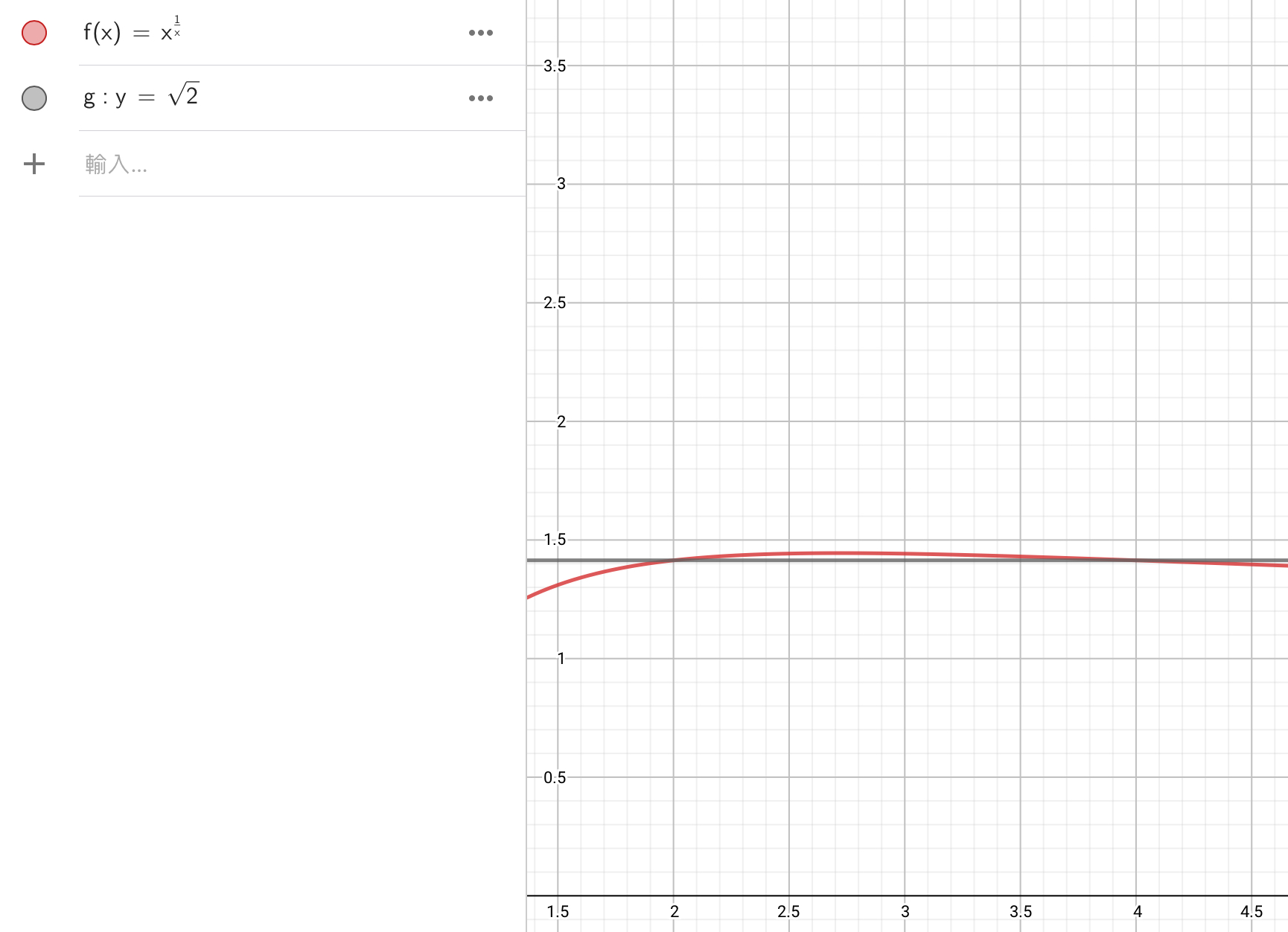e.g. the graphs intersect at $(2,\sqrt2)$ & $(4,\sqrt2)$ so $2^{\frac12}=4^{\frac14}=\sqrt2.$

- 1 year, 1 month ago

Two diferents values has same image :

other ejemple : f(x) = x^2

x= -2 and x= 2 => f(x) = 4

Relation is f inverse f(x) = $\sqrt{x}$

for x= 4 => f(x) = + 2 and -2

- 1 year, 1 month ago

@Jeff Giff- See here, I have already written that

- 1 year, 1 month ago

Solution will exist only when $a \in (0, 1)$. Now, you can draw graphs for y = $a^x$ and y = x and find the solution.

- 1 year, 1 month ago

solution exists if $a∈(0,e^{\frac{1}{e}})$, I recalculated it just now

- 1 year, 1 month ago

Anything wrong in this graph?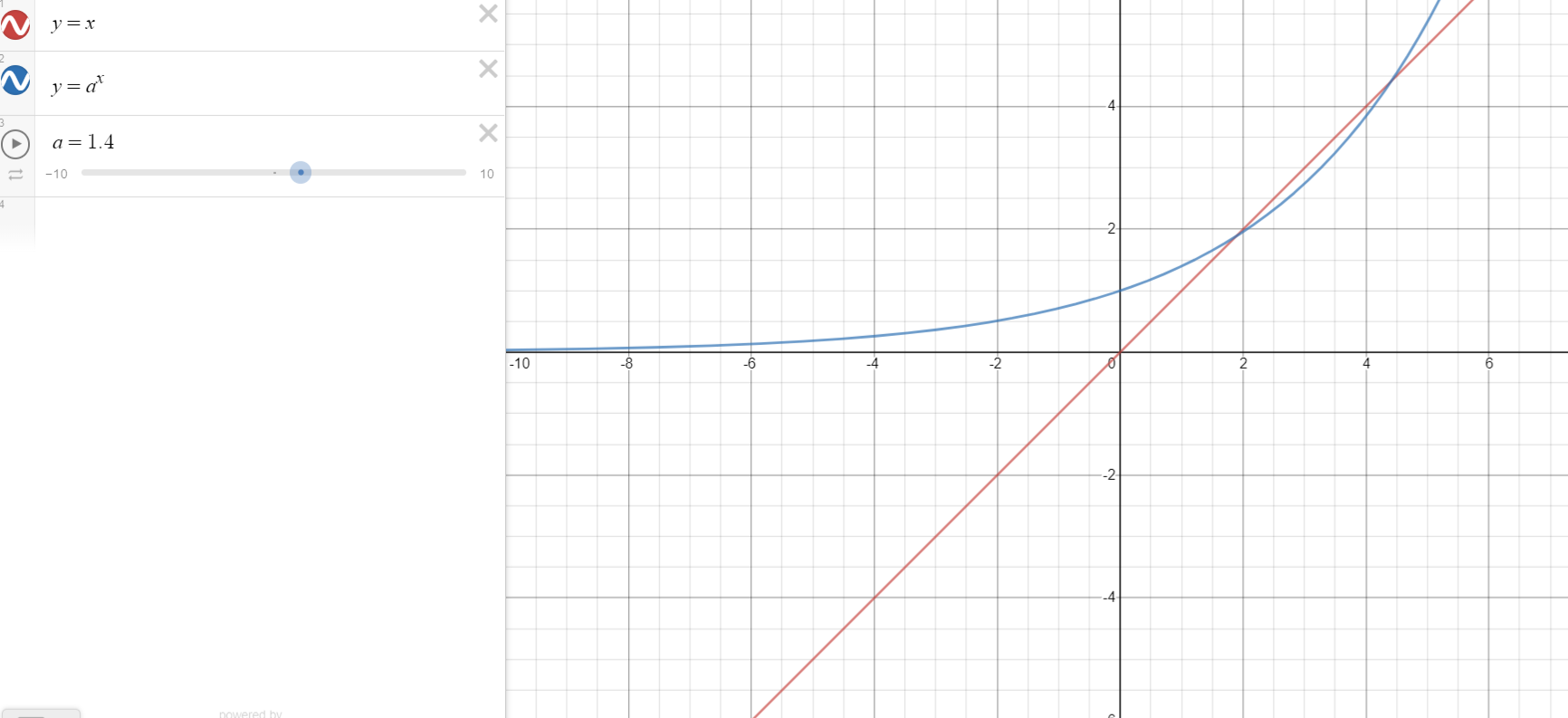- 1 year, 1 month ago

@Vinayak Srivastava - Nothing is wrong with the graph but, it is only for the case $a=1.4$ I want a general formula for the solution for any $a∈(0,e^\frac{1}{e})$

- 1 year, 1 month ago

@Aryan Sanghi- I am searching for a formula which gives value of $y$ for every value of $a$ where $a∈(0,e^\frac{1}{e})$

- 1 year, 1 month ago

$a \in (\frac{1}{e^e}, e^{\frac{1}{e}})$

- 1 year, 1 month ago

Well the $e^\frac{1}{e}$ comes by taking the maxima of the function $x^{1/x}$, but the minima is not $1/e^e$. If you know calculus and wanted proof for the given argument, then please comment.

- 1 year, 1 month ago

Sorry I haven't learned calculus

- 1 year, 1 month ago

Yes, you are right. I got same value as yours.

- 1 year, 1 month ago

@Chew-Seong Cheong, @Justin Travers, @jordi curto, @Yajat Shamji, @Mahdi Raza, @Kumudesh Ghosh, @Alak Bhattacharya, @Vinayak Srivastava, @Aryan Sanghi, @Jeff Giff, @Marvin Kalngan. For every $a∈(1,e^\frac{1}{e})$ there exists $2$ solutions for each $a$, one of them will be $y$ such that $y∈(1,e)$. I'm only considering that as solution for $1, for $0 there is only one solution for each $a$. Can anyone find any formula for these solutions where ($\red{0). I have also edited the note a bit, because I don't need $y>e$

- 1 year, 1 month ago

Why mention me? @Zakir Husain. I cannot do this stuff - please remove my mentions...

- 1 year, 1 month ago